• 4.8 微分方程微分方程是数值计算中常见的问题，MATLAB提供了多种函数来计算微分方程的解。4.8.1 常微分方程众所周知，对一些典型的常微分方程，能求解出它们的一般表达式，并用初始条件确定表达式中的任意常数。但...


4.8  微分方程微分方程是数值计算中常见的问题，MATLAB提供了多种函数来计算微分方程的解。4.8.1  常微分方程众所周知，对一些典型的常微分方程，能求解出它们的一般表达式，并用初始条件确定表达式中的任意常数。但实际中存在有这种解析解的常微分方程的范围十分狭窄，往往只局限在线性常系数微分方程(含方程组)，以及少数的线性变系数方程。对于更加广泛的、非线性的一般的常微分方程，通常不存在初等函数解析解。由于实际问题求解的需要，求近似的数值解成为了解决问题的主要手段。常见的求数值解的方法有欧拉折线法、阿当姆斯法、龙格-库塔法与吉尔法等。其中由于龙格-库塔法的精度较高，计算量适中，所以使用的较广泛。数值解的最大优点是不受方程类型的限制，即可以求任何形式常微分方程的特解(在解存在的情况下)，但是求出的解只能是数值解。1．龙格-库塔方法简介对于一阶常微分方程的初值问题，在求解未知函数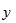时，在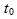点的值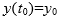是已知的，并且根据高等数学中的中值定理，应有：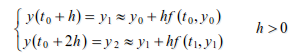一般而言，在任意点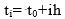，有：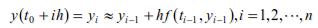当确定后，根据上述递推公式能算出未知函数在点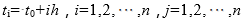的一列数值解：当然在递推的过程中同样存在着一个误差累计的问题，实际计算中的递推公式一般都进行过改造，龙格-库塔公式为：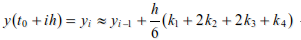其中：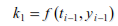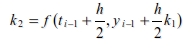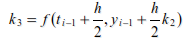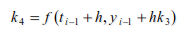2．龙格-库塔法的实现基于龙格-库塔法，MATLAB提供了ode系列函数求常微分方程的数值解。常用的有ode23 和ode45函数，其调用语法如下。(1)[t,y]=ode23(filename,tspan,y0)：采用了二阶、三阶龙格-库塔法进行计算。(2)[t,y]=ode45(filename,tspan,y0)：采用了四阶、五阶龙格-库塔法进行计算。其中filename是定义f(t,y)的函数文件名，该函数文件必须返回一个列向量。tspan的形式为[t0,tf]，表示求解区间。y0是初始状态列向量。t和y分别给出时间向量和相应的状态向量。这两个函数分别采用了二阶、三阶龙格-库塔法和四阶、五阶龙格-库塔法，并采用自适应变步长的求解方法，即当解的变化较慢时采用较大的步长，从而使得计算的速度很快；当解的变化较快时步长会自动地变小，从而使得计算的精度很高。【例4-45】  设有初值问题：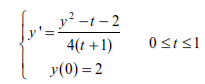试求其数值解，并和精确解相比较，精确解为(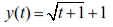)。首先要建立微分方程所对应的函数文件myodefun.m，文件内容如下：function y=myodefun(t,y)                      %  建立函数文件myodefun.my=(y^2-t-2)/(4*(t+1));建立myodefun函数之后，就可以调用ode23函数求解微分方程。>> t0=0;>> tf=10;>> y0=2;>> [t,y]=ode23 ('myodefun',[t0,tf],y0);       %  求数值解>> y1=sqrt(t+1)+1;                              %  求精确解>> plot(t,y,'k.',t,y1,'r')通过图形比较，数值解用黑色圆点表示，精确解用红色实线表示，如图4-13所示。【例4-46】  求下面无劲度系统微分方程组的数值解。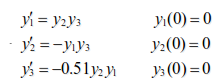为了求解方程，首先要建立方程的m文件。本例中不妨建立名为rigid.m的函数文件，此文件用以描述给出的方程组，文件的内容如下：function dy = rigid(t,y)dy = zeros(3,1);                 %  一个列向量dy(1) = y(2) * y(3);dy(2) = -y(1) * y(3);dy(3) = -0.51 * y(1) * y(2);本例中，我们通过odeset函数对误差进行控制，另外在时间[0 12]进行求解，0时刻初始条件向量为[0 1 1]。>> options =odeset('RelTol',1e-4,'AbsTol',[1e-4 1e-4 1e-5]);%  误差控制>> [T,Y] = ode45(@rigid,[0 12],[0 11],options);               %  求数值解>>plot(T,Y(:,1),'-',T,Y(:,2),'-.',T,Y(:,3),'.')               %  绘制结果图得到的结果如图4-14所示。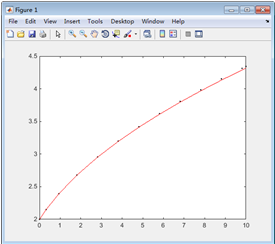图4-13  常微分方程结果图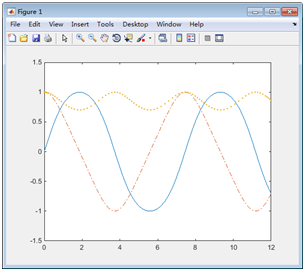图4-14  常微分方程数值展开全文• 【作者声明】　本文所有文字均为作者原创，所有图片均为作者本人亲自拍摄或制作。　版权所有，仅供阅读欣赏，禁止任何单位或个人以任何形式对...前言　我们知道，fx-991CN X计算器的“方程/函数”模式、SOLVE功能都...
【作者声明】　　本文所有文字均为作者原创，所有图片均为作者本人亲自拍摄或制作。　　版权所有，仅供阅读欣赏，禁止任何单位或个人以任何形式对本文的文字或图片进行包括但不限于复制、转载、引用、抄袭、截图、模仿、翻译、印刷等之中的一项或多项的行为。禁止将本文用于商业用途。　　作者保留所有权利，请尊重作者的劳动成果，谢谢合作。前言　　我们知道，fx-991CN X计算器的“方程/函数”模式、SOLVE功能都可以用来求解多项式方程，前者可以求解标准形式的一元二~四次方程，后者使用牛顿迭代法（切线法）求解。但是这两个功能都有局限性，就是不能求解系数为复数的方程。而且在fx-991CN X的复数模式中，SOLVE功能是无法使用的，因此我们必须想办法利用现有的功能来求解。数学基础与功能预备知识牛顿迭代公式：在计算模式下，SOLVE功能就是使用这个公式求解的，我们只需要输入方程，再指定一个初始值，计算器就能够按照初始值来计算方程的一个根。例如求解方程，我们只需要按[菜单]、进入计算模式，输入方程，按[SHIFT]、[CALC]进入SOLVE功能，指定一个迭代初始值（例如5）按[=]确认，再次按下[=]即可得到一个解“x=3”。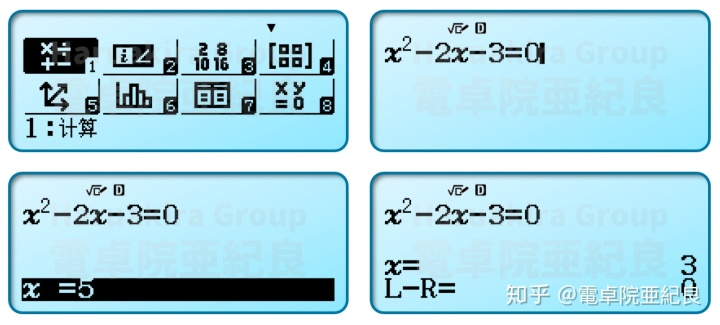如果不是很清楚SOLVE功能的使用，具体可以参考以前的这篇文章：電卓院亜紀良：fx-991CN X方程（组）求解功能的使用​zhuanlan.zhihu.com复数模式：按[菜单]、进入复数模式，可以进行复数的四则运算、整数次幂、共轭复数、实部/虚部提取、代数形式(a+bi)-极坐标形式(r∠θ)相互转换等等。如果不太了解复数功能，具体可以参考以前的这篇文章：電卓院亜紀良：使用科学计算器计算复数与相量（基础篇）​zhuanlan.zhihu.com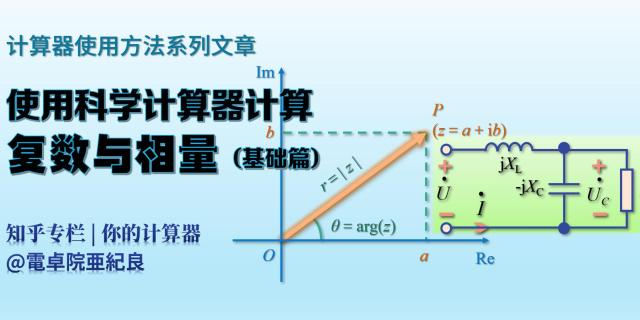在复数模式下求解复系数多项式方程　　在复数模式中，虽然SOLVE功能不能使用，但CALC功能仍然可以使用，那么我们可以使用CALC功能输入迭代式，间接求解复系数的多项式方程。【例】求解复系数方程【解】根据牛顿迭代公式，我们需要计算出函数的导数，多项式函数的导数是很容易计算的。这里给的是一个标准形式的多项式方程，如果遇到的多项式方程不是标准形式，最好先经过手算变形整理成标准形式。那么的导数就是所以迭代公式就是在计算器上就是输入按[菜单]、进入复数模式，输入上述迭代式，注意等号按[ALPHA]、[CALC]输入红色的等号，然后按[CALC]。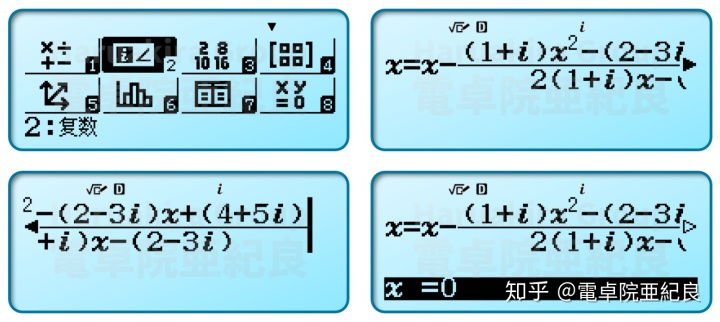这里需要输入迭代的初始值，一般输入一个实数即可，注意不要让原方程对应的函数的导数值为0，否则会出现数学错误。这里就以0为初始值，按[=]即可开始迭代；如果想要使用其他值作为初始值，那么就再输入一个数，按[=]确认之后，再按[=]开始迭代。迭代的时候会交替显示迭代当前值与赋值界面。按多次[=]后，即可发现x的值逐渐趋于不变，这时候即可认为已经迭代到了一个根：按[S-D]可以将实部和虚部显示为两行：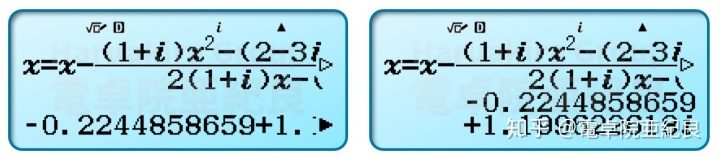如果要得到其他的根，在迭代完成的时候继续按[=]进入赋值界面，重新指定一个初始值，例如这里指定的是：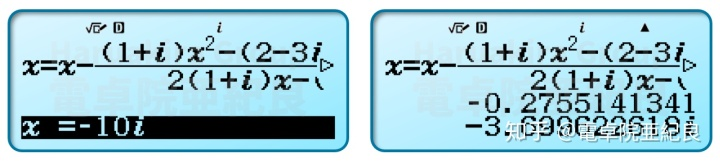这样，这个复系数一元二次方程就解出来了：后记　　这篇文章书写起因是一个提问，有关复系数方程求解的问题不止一次出现过，虽然带有CAS的图形计算器能够直接求解，但在只能使用fx-991CN X这样的计算器的考试中，利用现有的工具，加以简单的手算，搭配一些算法，就可以轻松地解决计算器不能直接求解的问题。另外还要感谢 @朽木家的优姬 、 @小林露露 的讨论与支持。
展开全文• 使用MATLAB求解方程求根——学习笔记 碎碎念：终于参加完了某比赛，连续大约摸了两天的鱼，就在昨天由于...求方程的根 法一： 求方程的全部根。  在MATLAB命令窗口输入：  p=[1,7,0,9,-20]; %建立多项式系数向...
使用MATLAB求解方程求根——学习笔记

碎碎念：终于参加完了某比赛，连续大约摸了两天的鱼，就在昨天由于自己的操作失误，亲手将电脑给烧了，这就是上天在暗示我是时候加油为接下来的两场比赛和一个考试努力啦~下面浅谈一下MATLAB各种求解方程的根的方法。

求方程的根

法一：

求方程的全部根。
在MATLAB命令窗口输入：
p=[1,7,0,9,-20];   %建立多项式系数向量
x=roots(p)         %求根

法二：

使用solve函数：

s=solve(f,v)：求方程关于指定自变量的解；

s=solve(f)：求方程关于默认自变量的解。

其中 f可以是用字符串表示的方程，或符号表达式；若f 中不含等号，则表示解 方程 f=0。

解方程 x^3-3*x+1=0

>>syms x; f=x^3-3*x+1;

>>s=solve(f,x)

>>s=solve(‘x^3-3*x+1’,‘x’)

>>s=solve(‘x^3-3*x+1=0’,‘x’)

Solve函数还可以用来求解方程组！

solve(f1,f2,...,fN,v1,v2,...,vN)

求解由 f1,f2,...,fN 确定的方程组关于 v1, v2,...,vN 的解。

解方程组：

>>[x,y,z]=solve(‘x+2*y-z=27’,‘x+z=3’, ...
‘x^2+3*y^2=28’,‘x’,‘y’,‘z’)

注意：输出变量的顺序要书写正确！

Solve在得不到解析解时，会给出数值解。

非线性方程组求解：

fzero(f,x0)：求方程f=0在x0附近的根。

几点说明：

方程可能有多个根，但是fzero只能给出距离x0最近的一个根；
若x0是一个标量，则fzero先找出一个包含x0的区间，使得f在这个区间两个端点上的值异号，然后再在这个区间内寻找方程f=0的根；如果找不到这样的区间，则返回NaN。
若x0是一个2维向量，则表示在[x0(1),x0(2)]区间内求方程的根，此时必须满足f在这两个端点上的值异号。
由于fzero是根据函数是否船业横轴来决定零点。因此它无法确定函数曲线仅触及横轴但是不穿越的零点，如|sin(x)|的所有零点。
函数中的f是一个函数句柄，通过以下方式给出：
字符串形式：fzero(‘x^3-3*x+1’,2);

通过@调用的函数句柄：fzero(@sin,4);

f不能用符号表达式！

线性方程组求解：

linsolve(A,b)：解线性方程组

>> A=[1 2 –1; 1 0 1; 1 3 0];

>> b=[2;3;8];

>> X=linsolve(A,b)

注意这里的b是列向量。

求解方程函数小结

roots(p)：多项式的所有零点，p是多项式系数向量。

fzero(f,x0)：求f=0在x0附近的根，f是函数句柄，可以由字符串给出或使用@， 但不能是符号表达式！

solve(f,v)：求方程关于指定自变量的解，f可以是用字符串表示的方程，或符号表 达式，若不含等号表示f=0；

也可解方程组(包含非线性)；得不到解析解时，给出数值解。

linsolve(A,b)：解线性方程组。
展开全文• 一阶线性常系数差分方程;Matlab实现 Xn (1+r^n X0;2. 建立M脚本文件 在M文件编辑器中输入 X0=100; r=0.0194; n=20; Xn=(1+r^n*X0 单击工具栏中的保存按钮文件名sqh1.m保存以后选择Debug:run菜单则会在指令窗口输出 >...
• ## 【matlab】解方程组

万次阅读 多人点赞 2018-02-01 11:33:55
例如，对于代数方程组Ax=b(A为系数矩阵，非奇异)的求解，MATLAB中有两种方法： (1)x=inv(A)*b — 采用逆运算解方程组； (2)x=A\b — 采用左除运算解方程组。 例: x1+2x2=8  2x1+3x2=13 >>A=[1,2;2,3];b...
在matlab中解方程组是很方便的
例如，对于代数方程组Ax=b(A为系数矩阵，非奇异)的求解，MATLAB中有两种方法：
(1)x=inv(A)*b — 采用求逆运算解方程组；
(2)x=A\b — 采用左除运算解方程组。
例:
x1+2x2=8
2x1+3x2=13
>>A=[1,2;2,3];b=[8;13];
>>x=inv(A)*b
x =
2.00
3.00
>>x=A\b
x =
2.00
3.00；
即二元一次方程组的解x1和x2分别是2和3。
对于同学问到的用matlab解多次的方程组，有符号解法，方法是：先解出符号解,然后用vpa(F,n)求出n位有效数字的数值解.具体步骤如下：
第一步:定义变量syms x y z ...;
第二步:求解[x,y,z,...]=solve('eqn1','eqn2',...,'eqnN','var1','var2',...'varN');
第三步:求出n位有效数字的数值解x=vpa(x,n);y=vpa(y,n);z=vpa(z,n);...。
如：解二（多）元二（高）次方程组：
x^2+3*y+1=0
y^2+4*x+1=0
解法如下：
>>syms x y;
>>[x,y]=solve('x^2+3*y+1=0','y^2+4*x+1=0');
>>x=vpa(x,4);
>>y=vpa(y,4);
结果是：
x =
1.635+3.029*i
1.635-3.029*i
-.283
-2.987
y =
1.834-3.301*i
1.834+3.301*i
-.3600
-3.307。
二元二次方程组，共4个实数根；
还有的同学问，如何用matlab解高次方程组（非符号方程组）？举个例子好吗？
解答如下：
基本方法是：solve(s1,s2,…,sn,v1,v2,…,vn)，即求表达式s1,s2,…,sn组成的方程组，求解变量分别v1,v2,…,vn。
具体例子如下：
x^2 + x*y + y = 3
x^2 - 4*x + 3 = 0
解法：
>> [x,y] = solve('x^2 + x*y + y = 3','x^2 - 4*x + 3 = 0')
运行结果为
x =
1 3
y =
1 -3/2
即x等于1和3；y等于1和-1.5
或
>>[x,y] = solve('x^2 + x*y + y = 3','x^2 - 4*x + 3= 0','x','y')
x =
1  3
y =
1 -3/2
结果一样，二元二方程都是4个实根。
通过这三个例子可以看出，用matlab解各类方程组都是可以的，方法也有多种，只是用到解方程组的函数，注意正确书写参数就可以了，非常方便。
用Matlab解方程组的时候，发现它不能自动代入系数的值。比如
说如下的程序;
a=4;
x=solve('a*x=4',x);
怎么解决？下面是解决办法，很简单。
法1：
symsa x b;
b = solve('a*x=4', x);
a = 4;
b=eval(b);
法2：
symsa x b;a = 4;
b = solve('a*x=4', x);
b=subs(b)

展开全文• Matlab多项式运算与方程求Matlab多项式运算 多项式四则运算 多项式四则运算续 多项式的导数polyder 多项式值 多项式值续 多项式根 注以上多项式运算中使用的都是多项式 的 系数向量不涉及符号计算 * * 在 ...
• 方程根 1.一元方程 y=x⋅sin(x)−x=0 y=x\cdot{sin(x)}-x=0 y=x⋅sin(x)−x=0 syms x; y = x*sin(x)-x; solve(y, x); 一元多项式方程可利用roots§求解，其中p为多项式系数 2.二元方程 {x−2y=5x+y=6 \begin{...
• 方程根 1.syms x--标明变量x是一个符号变量 2.solve(y,x)--y=0时，x的取值,默认...解单个方程(系数已知)： >> syms s >> y = s*sin(s)-s; >> solve(y,s) ans = 0 pi/2 >> ...
• ## matlab解方程、方程组

万次阅读 多人点赞 2016-06-23 17:11:03
最近有多人问如何用matlab方程组的问题，其实在matlab中解方程组还是很方便的，例如，对于代数方程组Ax=b(A为系数矩阵，非奇异)的求解，MATLAB中有两种方法： (1)x=inv(A)*b — 采用逆运算解方程组；  (2)x=A...
• ## MATLAB解线性方程组

千次阅读 2019-09-22 09:14:53
可以用来解线性方程组，矩阵的秩，矩阵行最简形（每行首元所在的列只有它一个是1）首元所在的列数。 例如 我们知道一个方程组 A*X=b 中 A 系数矩阵 和b列向量 A=[2 -2 2 6;2 -1 2 4; 3 -1 4 4;1 1 -1 3]; b=...
• 1、根据数学理论常系数线性微分方程可以求解解析解要想解析解可以用dsolve（）函数如果有初始值，则可以在dsolve函数参数funm后边添加初始值参数！2、其他微分方程可以求解数值解一般数值解可以利用ode45（），...
• MATLAB求解线性方程组的八种方法 求解线性方程分为两种方法–直接法和迭代法 常见的方法一共有8种 ...Gauss消去法 ：求解中小规模线性方程（阶数不过1000），一般用于求系数矩阵稠密而且没有任何特殊结构的线性方算法
• 我们将线性方程的求解分为两类：一类是方程唯一解或特解，另一类是方程无穷解即通解。可以通过系数矩阵的秩来判断：若系数矩阵的秩r=n(n为方程组中未知变量的个数)，则有唯一解；若系数矩阵的秩r，则可能...
• ## matlab 解方程组

万次阅读 多人点赞 2019-09-23 16:52:36
1、解方程最近有多人问如何用matlab方程组的问题，其实在matlab中解方程组还是很方便的，例如，对于代数方程组Ax=b(A为系数矩阵，非奇异)的求解，MATLAB中有两种方法：(1)x=inv(A)*b — 采用逆运算解方程组；...
• 其最直观的形式为 其中 和 都是正整数， 和 都是系数（ ， ），不妨设这些系数都属于复数域 ； 是未知数。如果取 以及 多元线性方程组可以写成 ，这里不再把矩阵和向量写成粗体。如果把 的列向量分别用 表示，即 ，...
• Matlab 由状态方程求系统传递函数矩阵代码：  已知状态方程系数矩阵如下： syms s GG gg; A=[0 1 0;0 0 1;-3 -1 -2]; B=[1 0; 0 1;1 1]; C=[1 1 1]; 利用自控原理的定理出传递函数的一般式： GG=C*inv...函数 程序
• MATLAB求解方程(1)roots函数功能：用于求解多项式...示例：求方程3x2-2x-4=0的根。代码：p=[3,-2,-4];r=roots(p);方程的根为x1=1.5352，x2=-0.8685.(2)solve函数功能：用于求解方程的符号解。调用格式：r=solve(eq...
• P = ploy(r)：以向量 r 内的元素为根，返回多项式的系数 P = ploy(A)：返回矩阵 A 的特征多项式 如果紧接着 roots(P) 的话可以得到矩阵 A 的特征值，与 eig(A) 等价 poly2str函数：poly2str(p,自变量)：返回...
• 如何利用C语言二元一次方程的解今天，小编我来详细的讲解一节C语言的具体运用。我们今天先拿二元一次方程来开刀。大家都知道二元一次方程的解，有三种情况，即无解， 有两个相同的解，有两个不同的解。那么在编程...
• PAGE / NUMPAGES 1解方程 最近有多人问如何用matlab方程组的问题其实在matlab中解方程组还是很方便的例如对于代数方程组Ax=b(A为系数矩阵非奇异)的求解MATLAB中有两种方法 (1)x=inv(A*b 采用逆运算解方程组 (2)x...
• 用矩阵函数求解一阶线性常系数齐次微分方程组主要步骤1.问题形式2.矩阵函数3.代入矩阵A的指数函数得最终解 主要步骤 本来想用在矩阵论期中开卷考试验证计算结果的，结果一个解方程组的题也没考…在一些学习网站白...算法
• 求解方程的根 eg.解方程x^2-x-2=0 1.roots( p )函数 此 MATLAB 函数 以列向量的形式返回 p 表示的多项式的根。...利用solve函数求方程的符号解，即求得的解是一个表达式，再利用eval函数计算表达...
• matlab线性方程组求解 ...对于非齐次线性方程组，可先由伪逆求方程组的一个特解，接着用null()函数求解线性方程组的解，其总解为特解加上某系数乘以线性解的和。 >> A=[3,2,1;1,-1,3;2,4,-4]; >> b
• 一：概述本篇文章介绍解线性方程组的平方根法及改进平方根法，适用范围为系数矩阵为正定Hermite矩阵（下称H阵）的线性方程组。这个方法的理论依据我觉得是来自Schur引理的H阵结构定理，从这个角度我们就可以理解课程...
• 定解方程组 计算过程： ...矩阵系数中，逗号和分号的使用 ... 未知数大于方程数，其解有无数个，matlab可以出其中一个特定解 计算过程 转载于:https://www.cnblogs.com/derek32/p/4042829.html...
• 一、定解方程组计算过程：注意：矩阵系数中，逗号和分号的使用矩阵除法用反斜杠\矩阵中的值提取用小括号，行列数从1开始算二、不定方程组未知数大于方程数，其解有无数个，matlab可以出其中一个特定解计算过程注意...
• %% 直接法线性方程组 % A=[2,1,-5,1;1,-5,0,7;0,2,1,-1;1,6,-1,-4];%系数 % b=[13,-9,6,0]'; % x=A\b %% 矩阵的分解线性方程组 %UL分解法 % A=[2,1,-5,1;1,-5,0,7;0,2,1,-1;1,6,-1,-4];%系数 % b=[13,-9,6,0]';...
• matlab求解差分方程程序 %差分方程为： %y(n)-2y(n-1)+3y(n-2)=4u(n)-5u(n-1)+6u(n-2)-7u(n-3) %初始条件：x(-1)=1,x(-2)=-1,y(-1)=-1,y(-2)=1,系统输出y(n) clear all; close all; clc; b=[4,-5,6,-7]; a=[1,-2,3...
• dsolve函数是matlab中自带的可以用来解决部分常微分方程的函数（方法），下面以物理学中的热传导问题为例展示如何使用这个函数。 热力学模型假设物体和恒温的环境接触，温度随时间的变化率和温差成正比，我们假设......

# matlab系数求方程matlab 订阅